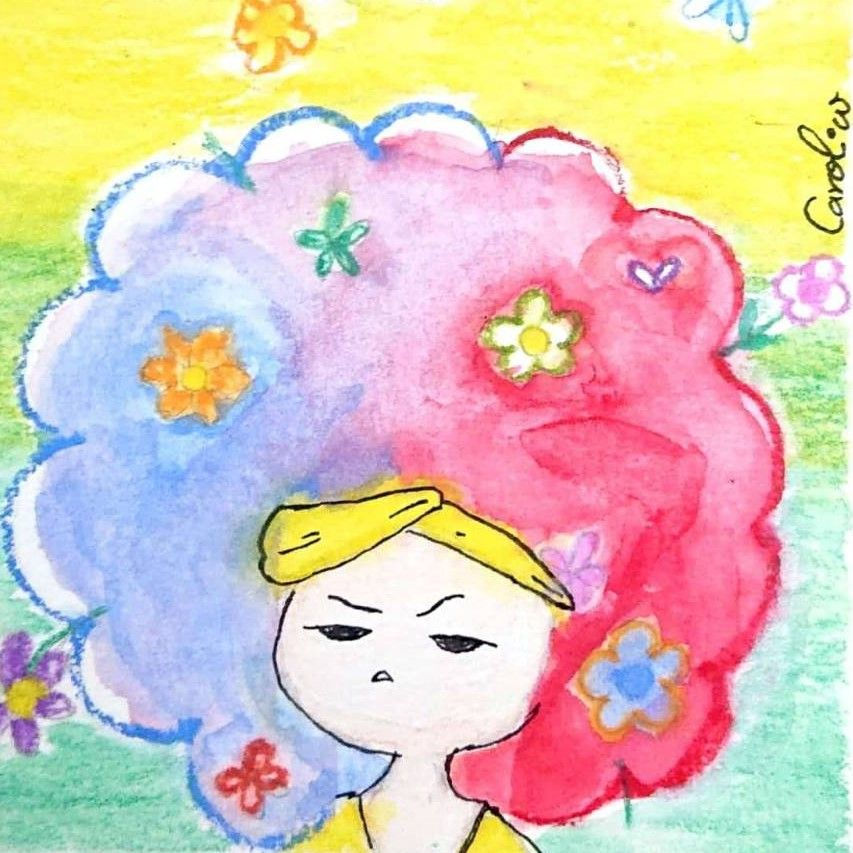Carol.WCarol.W

# 【畫話接力】｜看圖說故事 /回家路上<<結案報告>>🎉

(edited)
【畫話接力】｜看圖說故事 /回家路上<<結案報告>>🎉

```獎金分配：

4,488/23=195.13 ➡️ @分， 195 Likecoin.
Carol獨家 ➡️ @文，30 Likecoin
```

(獎金：195+30=225)

(獎金：195+30=225)

# 03.﻿@coco﻿ ，回家路上 / 淨灘

(圖自繪，獎金：195+195+30=420)

(獎金：195+30=225)

# 05.﻿@Angela Chen﻿ ，回家路上，觀音來作伴

(圖自繪，獎金：195+195+30=420)

(獎金：195+30=225)

(獎金：195+30=225)

(獎金：195+30=225)

(獎金：195+30=225)

# 10.﻿@Sogni﻿ ，回家路上– 徵文看圖說故事/回家路上

“此身如傳舍，何處是吾鄉？ 倒計時開始了嗎？“
“我生本無鄉，心安是吾鄉。 是的，我們會帶你回家。 “

(圖自繪，獎金：195+195+30=420)

(獎金：195+30=225)

(獎金：195+30=225)

(獎金：195+30=225)

(獎金：195+30=225)

(獎金：195+30=225)

(獎金：195+30=225)

# 17.﻿@毛毳﻿ ，【畫話接力】回家路上

(圖自繪，獎金：195+195+30=420)

(獎金：195+30=225)

# 19.﻿@莎茈﻿ ，【畫話接力】看圖說故事：那一年，楓樹下，橘貓遇見了她。

(獎金：195+30=225)

## 🥰再次謝謝大家的參與~~ 我們期待下個活動再相遇喔~🥰

⚠️ 本活動圖片作者為：﻿@奇多怪獸﻿ . ﻿@Carol.W﻿

♭♬ Carol😘: 悅 耳、歡快的歌~❤️♫♮

CC BY-NC-ND 2.0

Like my work?
Don't forget to support or like, so I know you are with me..